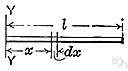# moment of inertia

Also found in: Thesaurus, Acronyms, Encyclopedia, Wikipedia.
Related to moment of inertia: Radius of gyration, Area moment of inertia

## moment of inertia

n. pl. moments of inertia
A measure of a body's resistance to angular acceleration, equal to:
a. The product of the mass of a particle and the square of its distance from an axis of rotation.
b. The sum of the products of each mass element of a body multiplied by the square of its distance from an axis.
c. The sum of the products of each element of an area multiplied by the square of its distance from a coplanar axis.
American Heritage® Dictionary of the English Language, Fifth Edition. Copyright © 2016 by Houghton Mifflin Harcourt Publishing Company. Published by Houghton Mifflin Harcourt Publishing Company. All rights reserved.

## moment of inertia

n
(General Physics) the tendency of a body to resist angular acceleration, expressed as the sum of the products of the mass of each particle in the body and the square of its perpendicular distance from the axis of rotation. Symbol: I
Collins English Dictionary – Complete and Unabridged, 12th Edition 2014 © HarperCollins Publishers 1991, 1994, 1998, 2000, 2003, 2006, 2007, 2009, 2011, 2014

## mo′ment of iner′tia

n.
the sum of the products of the mass and the square of the perpendicular distance to the axis of rotation of each particle in a body rotating about an axis.
[1820–30]
Random House Kernerman Webster's College Dictionary, © 2010 K Dictionaries Ltd. Copyright 2005, 1997, 1991 by Random House, Inc. All rights reserved.
ThesaurusAntonymsRelated WordsSynonymsLegend:
 Noun 1moment of inertia - the tendency of a body to resist angular accelerationinertia - (physics) the tendency of a body to maintain its state of rest or uniform motion unless acted upon by an external forcemoment - a turning force produced by an object acting at a distance (or a measure of that force)
Based on WordNet 3.0, Farlex clipart collection. © 2003-2012 Princeton University, Farlex Inc.
References in periodicals archive ?
A steel disc of radius [r.sub.2] , mass [m.sup.2] and central moment of inertia [J.sub.G2] is mounted coaxially to a brass bushing of negligible mass, into which a cylindrical hole of radius r is made.
With a 1000mm arm length, the allowable moment of inertia for the THL1000 is 0.2kg/m2; and by using absolute encoders, the robots' repeatability in X-Y is 0.01mm.
The dynamic track properties of the turnout model such as rail masses' pitch moment of inertia, railpads' stiffness and damping, and ballast's stiffness and damping are validated beforehand by comparing the receptance functions from a straight track model to the receptance functions recorded in the literature.
Then, a reference value for the normalized cutoff frequency ([[lambda].sub.r]), for the axis corresponding to the mean principal moment of inertia ([J.sub.r]), can be determined through numerical simulations, assuming [f.sub.co] is equal to the maximum angular rate [[omega].sub.max] that can be expected at the deployment (this value is eventually provided by the deployer datasheet or can be guessed during mission analysis, depending on the geometry of the satellite and on the characteristics of the deployer).
where [I.sub.em] and [I.sub.ec] are the effective moment of inertia at the midspan and at the middle support, respectively.
The best accuracy can be achieved by the NFC technique, and the 3 image feature variables are area, moment of inertia, and perimeter.
If the increase the moment of inertia of the electric drive, then the IM will operate longer at reduced speeds, where the coefficients of attenuation of the stator and rotor circuits are small, which determines slower ET attenuation with increase in the total moment inertia [J.sub.[SIGMA]] of the electric drive and the mechanism.
[J.sub.2] - Polar moment of inertia of the hollow shaft
However, these outlines would fail to explain IB's at low spin, where the blocking of the pairing contributions of the odd nucleon is predicted to reduce the nuclear superfluidity, there by increasing the moment of inertia of the odd-A nucleus.

Site: Follow: Share:
Open / Close